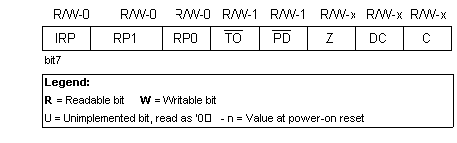# Central Processing Unit

Central processing unit (CPU) is the brain of a microcontroller. That part is responsible for finding and fetching the right instruction which needs to be executed, for decoding that instruction, and finally for its execution.

Central processing unit connects all parts of the microcontroller into one whole. Surely, its most important function is to decode program instructions. When programmer writes a program, instructions have a clear form like MOVLW 0x20. However, in order for a microcontroller to understand that, this 'letter' form of an instruction must be translated into a series of zeros and ones which is called an 'opcode'. This transition from a letter to binary form is done by translators such as assembler translator (also known as an assembler). Instruction thus fetched from program memory must be decoded by a central processing unit. We can then select from the table of all the instructions a set of actions which execute a assigned task defined by instruction. As instructions may within themselves contain assignments which require different transfers of data from one memory into another, from memory onto ports, or some other calculations, CPU must be connected with all parts of the microcontroller. This is made possible through a data bus and an address bus.

Arithmetic logic unit is responsible for performing operations of adding, subtracting, moving (left or right within a register) and logic operations. Moving data inside a register is also known as 'shifting'. PIC16F84 contains an 8-bit arithmetic logic unit and 8-bit work registers.

In instructions with two operands, ordinarily one operand is in work register (W register), and the other is one of the registers or a constant. By operand we mean the contents on which some operation is being done, and a register is any one of the GPR or SFR registers. GPR is an abbreviation for 'General Purposes Registers', and SFR for 'Special Function Registers'. In instructions with one operand, an operand is either W register or one of the registers. As an addition in doing operations in arithmetic and logic, ALU controls status bits (bits found in STATUS register). Execution of some instructions affects status bits, which depends on the result itself. Depending on which instruction is being executed, ALU can affect values of Carry (C), Digit Carry (DC), and Zero (Z) bits in STATUS register.

## STATUS Registerbit 7
IRP (Register Bank Select bit)
Bit whose role is to be an eighth bit for purposes of indirect addressing the internal RAM.
1 = bank 2 and 3
0 = bank 0 and 1 (from 00h to FFh)

bits 6:5
RP1:RP0 (Register Bank Select bits)
These two bits are upper part of the address for direct addressing. As instructions which address the memory directly have only seven bits, they need one more bit in order to address all 256 bytes which is how many bytes PIC16F84 has. RP1 bit is not used, but is left for some future expansions of this microcontroller.
01 = first bank
00 = zero bank

bit 4
TO Time-out ; Watchdog overflow.
Bit is set after turning on the supply and execution of CLRWDT and SLEEP instructions. Bit is reset when watchdog gets to the end signaling that overflow took place.
1 = overflow did not occur
0 = overflow did occur

bit 3
PD (Power-down bit)
This bit is set whenever power supply is brought to a microcontroller : as it starts running, after each regular reset and after execution of instruction CLRWDT. Instruction SLEEP resets it when microcontroller falls into low consumption mode. Its repeated setting is possible via reset or by turning the supply off/on . Setting can be triggered also by a signal on RB0/INT pin, change on RB port, upon writing to internal DATA EEPROM, and by a Watchdog.
1 = after supply has been turned on
0 = executing SLEEP instruction

bit 2
Z (Zero bit) Indication of a zero result
This bit is set when the result of an executed arithmetic or  logic operation is zero.
1 = result equals zero
0 = result does not equal zero

bit 1
DC (Digit Carry) DC Transfer
Bit affected by operations of addition, subtraction. Unlike C bit, this bit represents transfer from the fourth resulting place. It is set in case of subtracting smaller from greater number and is reset in the other case.
1 = transfer occurred on the fourth bit according to the order of the result
0 = transfer did not occur

bit 0
C (Carry) Transfer
Bit that is affected by operations of addition, subtraction and shifting.
1 = transfer occurred from the highest resulting bit
0 = transfer did not occur